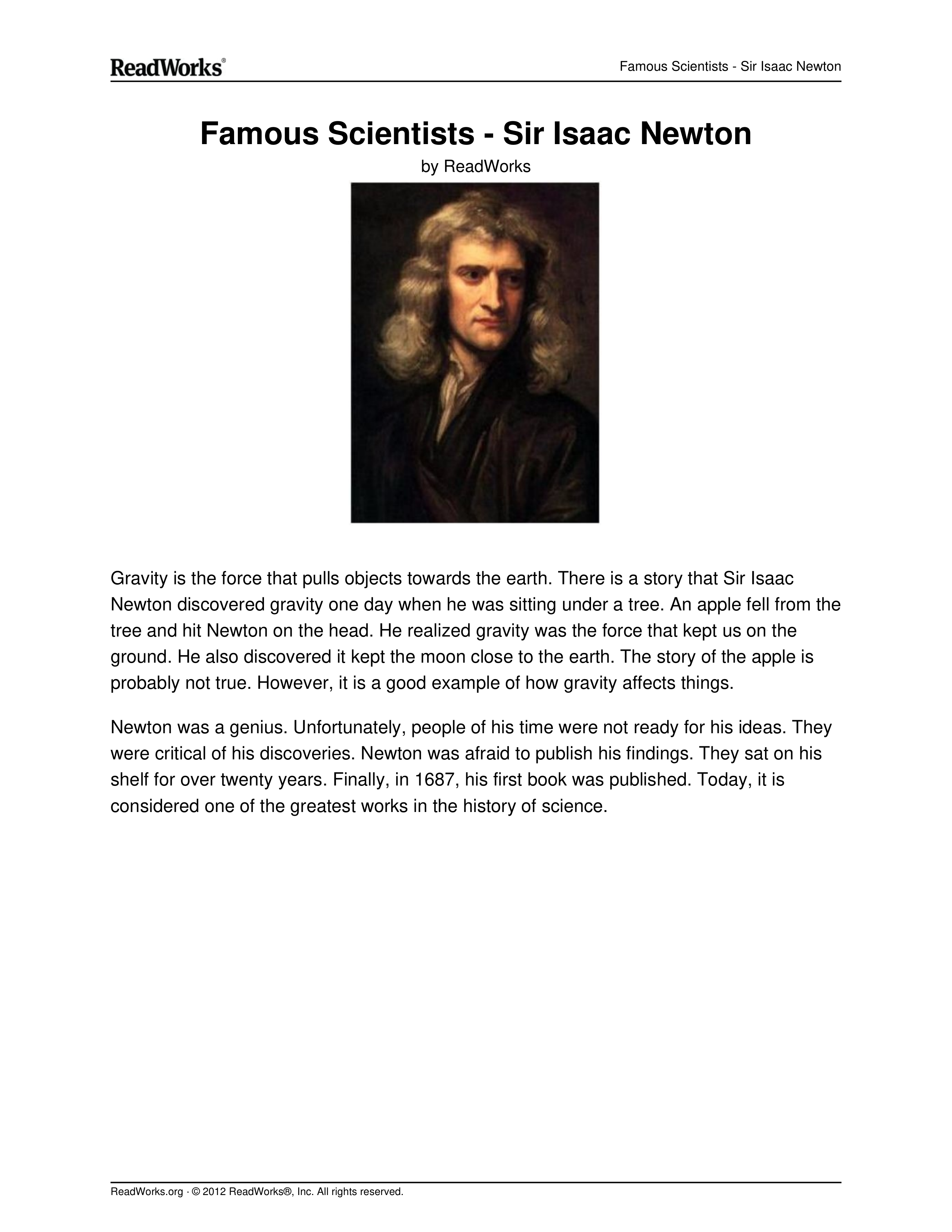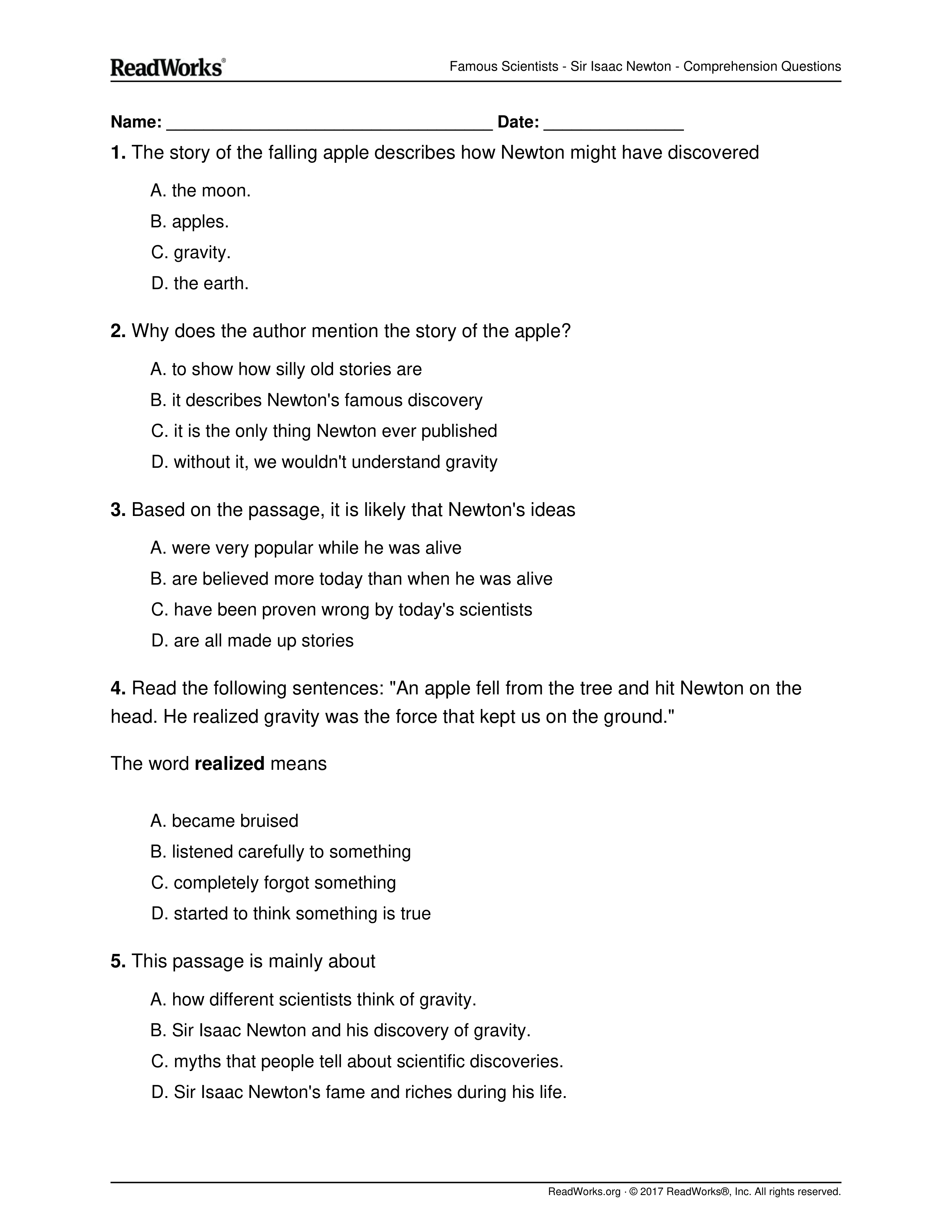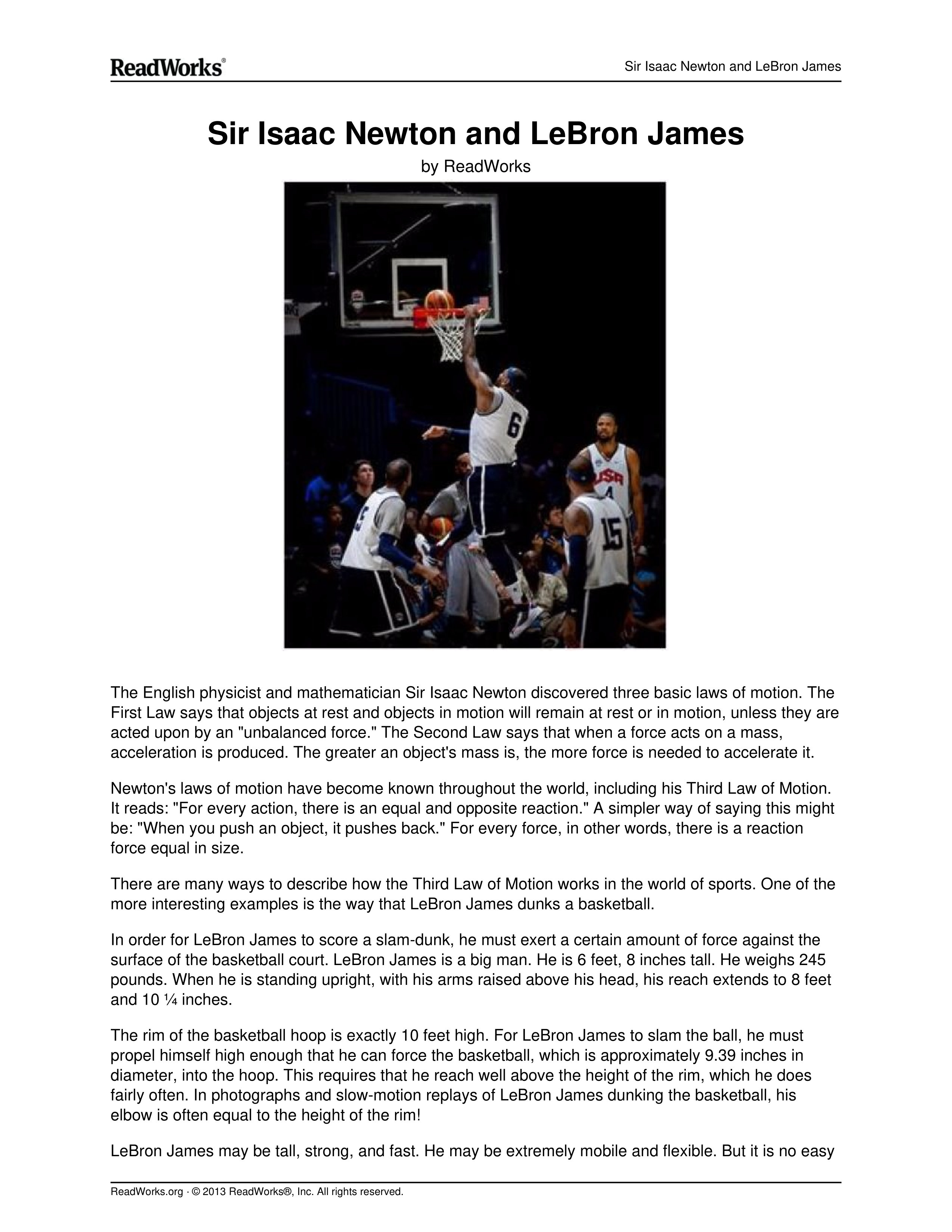Newton's Laws
starstarstarstarstarstarstarstarstarstar
by Sarah Peraza
| 20 Questions1
2
3
4
5
1
1
A
B
C
D
2
1
A
B
C
D
3
1
A
B
C
D
4
1
A
B
C
D
5
1
A
B
C
D6
7
8
9
6
1
7
1
8
1
A
B
C
D
9
1
Go to the vocabulary assign. Complete the vocabulary.com practice. Then continue Formative.
10
1
What word (s) did you learn that you did not know before?

# Newton's Laws of Motion

There was this fellow in England named Sir Isaac Newton. A little bit stuffy, bad hair, but quite an intelligent guy. He worked on developingcalculus and physics at the same time. During his work, he came up with the three basic ideas that are applied to the physics of most motion (NOTmodern physics). The ideas have been tested and verified so many times over the years, that scientists now call them Newton's Three Laws of Motion

# First Law

📷The first law says that an object at rest tends to stay at rest, and an object in motion tends to stay in motion, with the same direction and speed. Motion (or lack of motion) cannot change without an unbalanced force acting. If nothing is happening to you, and nothing does happen, you will never go anywhere. If you're going in a specific direction, unless something happens to you, you will always go in that direction. Forever.
You can see good examples of this idea when you see video footage of astronauts. Have you ever noticed that their tools float? They can just place them in space and they stay in one place. There is no interfering force to cause this situation to change. The same is true when they throw objects for the camera. Those objects move in a straight line. If they threw something when doing a spacewalk, that object would continue moving in the same direction and with the same speed unless interfered with; for example, if a planet's gravity pulled on it (Note: This is a really really simple way of descibing a big idea. You will learn all the real details - and math - when you start taking more advanced classes in physics.).

# Second Law

📷The second law says that theacceleration of an object produced by a net (total) applied force is directly related to the magnitude of the force, the same direction as the force, and inversely related to the mass of the object (inverse is a value that is one over another number... the inverse of 2 is 1/2). The second law shows that if you exert the same force on two objects of different mass, you will get different accelerations (changes in motion). The effect (acceleration) on the smaller mass will be greater (more noticeable). The effect of a 10 newton force on a baseball would be much greater than that same force acting on a truck. The difference in effect (acceleration) is entirely due to the difference in their masses.

# Third Law

The third law says that for every action (force) there is an equal and opposite reaction (force). Forces are found in pairs. Think about the time you sit in a chair. Your body exerts a force downward and that chair needs to exert an equal force upward or the chair will collapse. It's an issue of symmetry. Acting forces encounter other forces in the opposite direction. There's also the example of shooting a cannonball. When the cannonball is fired through the air (by the explosion), the cannon is pushed backward. The force pushing the ball out was equal to the force pushing the cannon back, but the effect on the cannon is less noticeable because it has a much larger mass. That example is similar to the kick when a gun fires a bullet forward.
11
1
Newton’s First Law is also known as the law of
A Inertia
B Motion
C Force
D Mass
12
1
Force is the product of ...
A Mass and charge
B Matter and energy
C Particles and waves
D Mass and acceleration
E Speed and weight
13
1
According to Newton's Third Law, for every action there is an equal and opposite
A force
B reaction
C push
D retaliation
E energization
Review the video you watched earlier. Answer questions that follow.
14
1
If an object is at rest, what must be applied to get it moving?
A force
B acceleration
C speed
D mass
E energy
15
1
Why would it be really difficult to pedal a 10,000 pound bicycle?
A To accelerate a large mass requires a large force
B Large masses tend to spontaneously stop
C The reaction of the bicycle to force is unfavorable
D Lighter objects require larger forces to accelerate quicker
E It would not be difficult16
1

#### What is Sir Isaac Newton’s Third Law of Motion?

A Objects at rest and objects in motion will remain at rest or in motion, unless they are acted upon by an unbalanced force.
B For every action there is an equal and opposite reaction.
C When a force acts on a mass, acceleration is produced.
D When a force acts on a mass, the mass increases.
17
1

#### What does the author describe in the passage?

A Sir Isaac Newton’s most famous book, Mathematical Principles of Natural Philosophy
B how LeBron James developed his basketball dunking skills
C how Sir Isaac Newton came up with the three basic laws of motion
D how the way that LeBron James dunks a basketball illustrates Newton’s Third Law of Motion
18
1

#### Read the following sentences from the passage: “When LeBron James jumps, he pushes down on the surface of the court. This is the 'action' that Newton mentions in his Third Law.”Based on this information, LeBron James jumping is an example of which part of Newton’s Third Law?

A both the action and the equal and opposite reaction
B the equal and opposite reaction of an action
C the action which causes an equal and opposite reaction
D neither the action nor the equal and opposite reaction
19
1

20
1